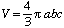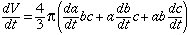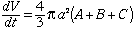hi im a student instructor in 14th grade i cant get this problem for my calc student coould you help me out as soon as possible The volume of an ellipsoid whose semiaxes are of the lengths a,b,and c is 4/3 *pi*abc. Suppose semiaxes a is changing at a rate of A cm/s , the semiaxes b is changing at B cm/s and the semiaxes c is changing at C cm/s . If the volume of the ellipsoid is decreasing when a=b=c what can you say about A,B,C? Justify. I really cant get it, does anyone know this???? Hi, The volume iswhere V, a, b and c are all functions of time, t. Thus, if you differentiate with respect to t you getIf you designate the rate of change of a, b and C as A, B and C respectively and if a = b = c thenSince the volume is decreasing the rate of change of V is negative so A + B + C is negative. Cheers, Harley Go to Math Central